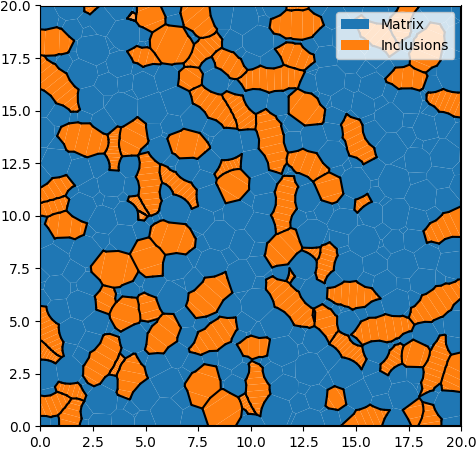# 1. Basic Example¶

## 1.1. XML Input File¶

The basename for this file is intro_1_basic.xml. The file can be run using this command:

microstructpy --demo=intro_1_basic.xml


The full text of the file is:

<?xml version="1.0" encoding="UTF-8"?>
<input>
<material>
<name> Matrix </name>
<material_type> matrix </material_type>
<fraction> 2 </fraction>
<shape> circle </shape>
<size>
<dist_type> uniform </dist_type>
<loc> 0 </loc>
<scale> 1.5 </scale>
</size>
</material>

<material>
<name> Inclusions </name>
<fraction> 1 </fraction>
<shape> circle </shape>
<diameter> 2 </diameter>
</material>

<domain>
<shape> square </shape>
<side_length> 20 </side_length>
<corner> (0, 0) </corner>
</domain>

<settings>
<verbose> True </verbose>
<directory> intro_1_basic </directory>
</settings>
</input>


## 1.2. Materials¶

There are two materials, in a 2:1 ratio based on volume. The first is a matrix, which is represented with small circles. The size and shape of matrix grain particles are not critical, since the boundaries between them will be removed before triangular meshing. The second material consists of circular inclusions with diameter 2.

## 1.3. Domain Geometry¶

The domain of the microstructure is a square with its bottom-left corner fixed to the origin. The side length is 20, which is 10x the size of the inclusions to ensure that the microstructure is statistically representative.

## 1.4. Settings¶

Many settings have been left to their defaults, with the exceptions being the verbose mode and output directory.

By default, MicroStructPy does not print status updates to the command line. Switching the verbose mode on will regularly print the status of the code.

The output directory is a filepath for writing text and image files. By default, MicroStructPy outputs texts files containing data on the seeds, polygon mesh, and triangular mesh as well as the corresponding image files, saved in PNG format.

Note

The <directory> field can be an absolute or relative filepath. If it is relative, outputs are written relative to the input file, not the current working directory.

## 1.5. Output Files¶

The three plots that this file generates are the seeding, the polygon mesh, and the triangular mesh. These three plots are shown in Fig. 1.1 - Fig. 1.3.Fig. 1.1 Introduction 1 - seed geometries.Fig. 1.2 Introduction 1 - polygonal mesh.Fig. 1.3 Introduction 1 - triangular mesh.